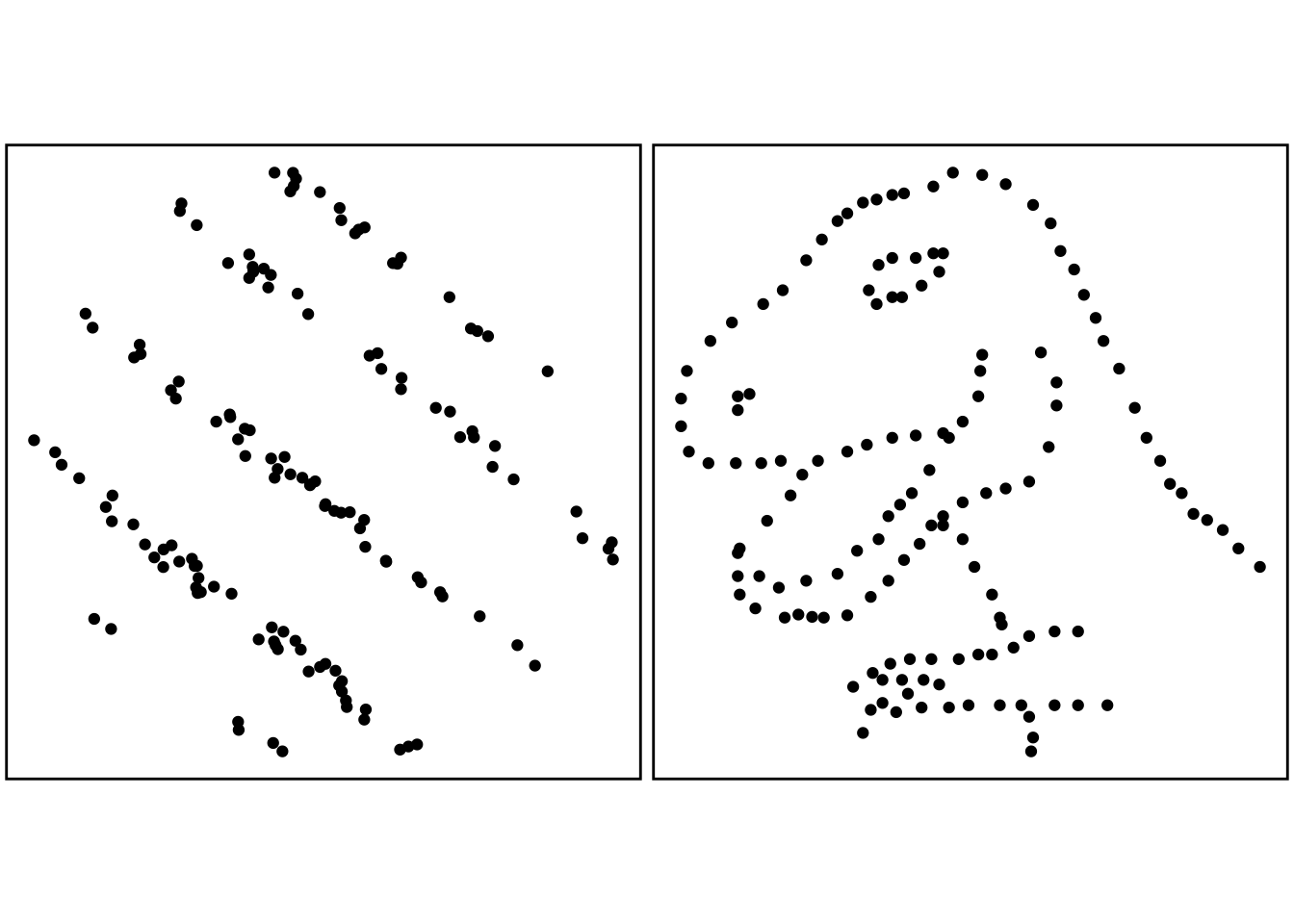Data are from the wonderful Datasaurus dozen, available from the Cran R package. They use Alberto Cairo’s Datasaurus data.

library(magrittr)
library(ggplot2)
library(ggpubr)
library(datasauRus)

slant <- ggplot(datasaurus_dozen_wide, aes(x=slant_down_x,y=slant_down_y),   colour=dataset) # loads slant-pattern dataset of datasauRus package into data frame slant
slant <- slant + geom_point() # as a scatter type geom
slant <- slant + theme_void() # eliminates unwanted axis labels
slant <- slant + theme(legend.position = "none", panel.border = element_rect(colour = "black", fill=NA, size = 1), plot.margin = margin(0,2,0,2), aspect.ratio = 1) # removes legend, adds a border, adds margin space below, and specifies required aspect ratio

dino <- ggplot(datasaurus_dozen_wide, aes(x=dino_x,y=dino_y), colour=dataset) +
geom_point() # loads dino-figure dataset of datasauRus package into data frame dino as a scatter type geom
dino <- dino +theme_void() # eliminates unwanted axis labels
dino <- dino + theme(legend.position = "none", panel.border =  element_rect(colour = "black", fill=NA, size = 1), plot.margin = margin(0,2,0,2), aspect.ratio = 1) # removes legend, adds a border, adds margin space below, specifies required aspect ratio

p <- ggarrange(slant, dino, ncol=2, nrow=1) # vertically frames the two plots

pFigure 2.6 Two sets of (fictitious) data-points for which the Pearson correlation coefficients are both 0. This clearly does not mean there is no relationship between the two variables being plotted.